# Bilateral Filtering¶

Important: Please read the installation page for details about how to install the toolboxes. $\newcommand{\dotp}{\langle #1, #2 \rangle}$ $\newcommand{\enscond}{\lbrace #1, #2 \rbrace}$ $\newcommand{\pd}{ \frac{ \partial #1}{\partial #2} }$ $\newcommand{\umin}{\underset{#1}{\min}\;}$ $\newcommand{\umax}{\underset{#1}{\max}\;}$ $\newcommand{\umin}{\underset{#1}{\min}\;}$ $\newcommand{\uargmin}{\underset{#1}{argmin}\;}$ $\newcommand{\norm}{\|#1\|}$ $\newcommand{\abs}{\left|#1\right|}$ $\newcommand{\choice}{ \left\{ \begin{array}{l} #1 \end{array} \right. }$ $\newcommand{\pa}{\left(#1\right)}$ $\newcommand{\diag}{{diag}\left( #1 \right)}$ $\newcommand{\qandq}{\quad\text{and}\quad}$ $\newcommand{\qwhereq}{\quad\text{where}\quad}$ $\newcommand{\qifq}{ \quad \text{if} \quad }$ $\newcommand{\qarrq}{ \quad \Longrightarrow \quad }$ $\newcommand{\ZZ}{\mathbb{Z}}$ $\newcommand{\CC}{\mathbb{C}}$ $\newcommand{\RR}{\mathbb{R}}$ $\newcommand{\EE}{\mathbb{E}}$ $\newcommand{\Zz}{\mathcal{Z}}$ $\newcommand{\Ww}{\mathcal{W}}$ $\newcommand{\Vv}{\mathcal{V}}$ $\newcommand{\Nn}{\mathcal{N}}$ $\newcommand{\NN}{\mathcal{N}}$ $\newcommand{\Hh}{\mathcal{H}}$ $\newcommand{\Bb}{\mathcal{B}}$ $\newcommand{\Ee}{\mathcal{E}}$ $\newcommand{\Cc}{\mathcal{C}}$ $\newcommand{\Gg}{\mathcal{G}}$ $\newcommand{\Ss}{\mathcal{S}}$ $\newcommand{\Pp}{\mathcal{P}}$ $\newcommand{\Ff}{\mathcal{F}}$ $\newcommand{\Xx}{\mathcal{X}}$ $\newcommand{\Mm}{\mathcal{M}}$ $\newcommand{\Ii}{\mathcal{I}}$ $\newcommand{\Dd}{\mathcal{D}}$ $\newcommand{\Ll}{\mathcal{L}}$ $\newcommand{\Tt}{\mathcal{T}}$ $\newcommand{\si}{\sigma}$ $\newcommand{\al}{\alpha}$ $\newcommand{\la}{\lambda}$ $\newcommand{\ga}{\gamma}$ $\newcommand{\Ga}{\Gamma}$ $\newcommand{\La}{\Lambda}$ $\newcommand{\si}{\sigma}$ $\newcommand{\Si}{\Sigma}$ $\newcommand{\be}{\beta}$ $\newcommand{\de}{\delta}$ $\newcommand{\De}{\Delta}$ $\newcommand{\phi}{\varphi}$ $\newcommand{\th}{\theta}$ $\newcommand{\om}{\omega}$ $\newcommand{\Om}{\Omega}$

This tour explores edge preserving filtering and in particular the bilateral filter, with applications to denoising and detail enhancement.

In :
warning off
warning on


## Gaussian Linear Filtering¶

The most basic filtering operation is the Gaussian filtering, that tends to blur edges.

Image size.

In :
n = 256*2;


In :
name = 'hibiscus';
f0 = rescale(crop( sum(f0,3) ,n));


A Gaussian filter of variance $\si$ reads $$\Gg_\si(f)(x) = \frac{1}{Z} \sum_y G_\si(x-y) f(y) \qwhereq Z = \sum_y G_\si(y),$$ and where the Gaussian kernel is defined as: $$G_\si(x) = e^{-\frac{\norm{x}^2}{2\si^2}}.$$

A convolution can be computed either over the spacial domain in $O(N\si_x^2)$ operations or over the Fourier domain in $O(N \log(N))$ operations. Depending on the value of $\si_x$, one should prefer either Fourier or spacial domain. For simplicity, we consider here the Fourier domain (and hence periodic boundary conditions).

Define a Gaussian function, centered at the top left corner (because it corresponds to the 0 point for the FFT).

In :
x = [0:n/2-1, -n/2:-1];
[Y,X] = meshgrid(x,x);
GaussianFilt = @(s)exp( (-X.^2-Y.^2)/(2*s^2) );


Display a recentered example of Gaussian.

In :
clf;
imageplot(fftshift(GaussianFilt(40)));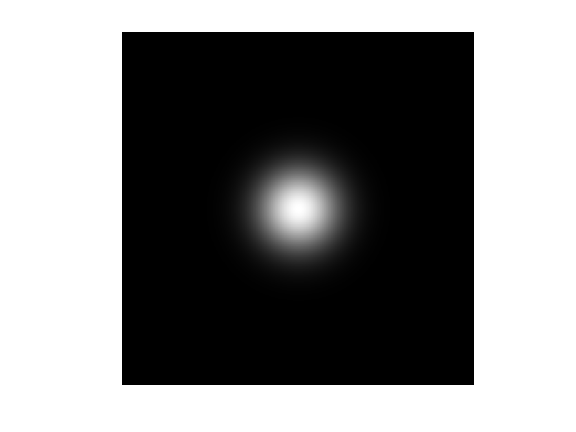Define a shortcut to perform linear Gaussian filtering over the Fourier domain. This function is able to process in parallel a 3D block |F| by filtering each |F(:,:,i)|.

In :
Filter = @(F,s)real( ifft2( fft2(F).*repmat( fft2(GaussianFilt(s)), [1 1 size(F,3)] ) ) );


Example of filtering $\Gg_\si(f_0)$.

In :
clf;
imageplot( Filter(f0,5) );Exercise 1

Display a filtering $\Gg_\si(f_0)$ with increasing size $\si$.

In :
exo1()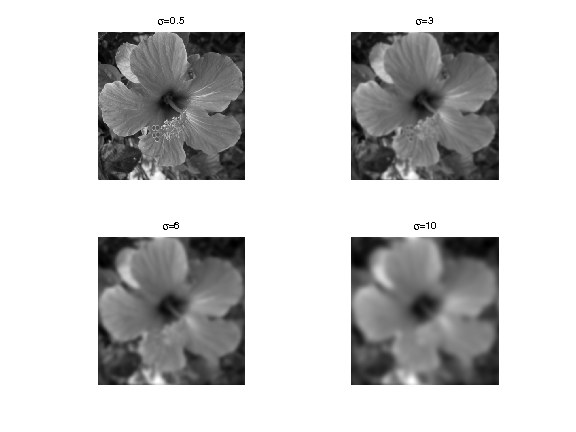In :
%% Insert your code here.


## Bilateral Filter¶

The bilateral filter is a spacially varying filter that better preserves edges than the Gaussian filter.

It was first introduced in:

Carlo Tomasi and Roberto Manduchi, Bilateral Filtering for Gray and Color Images, Proceedings of the ICCV 1998

A very good set of ressources concerning the bilateral filter can be found on <http://people.csail.mit.edu/sparis/bf/ Sylvain Paris web page>. It includes a fast implementation, research and review papers.

Given a spacial width $\si_x$ and a value width $\si_v$, the filter opterates as: $$\Bb_{\si_x,\si_v}(f)(x) = \frac{1}{Z_x} \sum_y G_{\si_x}( x-y ) G_{\si_v}(f(x)-f(y)) f(y)$$ where the normalizing constant is $$Z_x = \sum_y G_{\si_x}( x-y ) G_{\si_v}(f(x)-f(y)).$$

At a given pixel $x$, it corresponds to an averaring with the data-dependant kernel $G_{\si_x}( x-y ) G_{\si_v}(f(x)-f(y))$.

## Bilateral Filter by Stacking¶

Implementing the bilateral filter directly over the spacial domain requires $O( N \si_x^2 )$ operations where $N$ is the number of pixels.

A fast approximate implementation is proposed in:

Fast Bilateral Filtering for the Display of High-Dynamic-Range Images, Fredo Durand and Julie Dorsey, SIGGRAPH 2002.

It exploits the fact that for all pixels $x$ with the same value $v=f(x)$, the bilateral filter can be written as a ratio of convolution $$\Bb_{\si_x,\si_v}(f)(x) = F_v(x) = \frac{ [G_{\si_x} \star ( f \cdot W_v )](x) }{ [G_{\si_x} \star W_v](x) }$$ where the weight map reads $$W_v(x) = G_{\si_v}(v-f(x)).$$

Instead of computing all possible weight maps $W_v$ for all possible pixel values $v$, one considers a subset $\{v_i\}_{i=1}^p$ of $p$ values and computes the weights $\{ W_{v_i} \}_i$.

Using these convolutions, one thus optains the maps $\{ F_{v_i} \}_i$, that are combined to obtain an approximation of $\Bb_{\si_x,\si_v}(f)$.

The computation time of the method is $O(p N\log(N))$ over the Fourier domain (the method implemented in this tour) and $O(p N \si_x^2)$ over the spacial domain.

Value of $\sigma_x$:

In :
sx = 5;


Value of $\sigma_v$:

In :
sv = .2;


Number $p$ of stacks. The complexity of the algorithm is proportional to the number of stacks.

In :
p = 10;


Function to build the weight stack $\{ W_{v_i} \}_i$ for $v_i$ uniformly distributed in $[0,1]$.

In :
Gaussian = @(x,sigma)exp( -x.^2 / (2*sigma^2) );
WeightMap = @(f0,sv)Gaussian( repmat(f0, [1 1 p]) - repmat( reshape((0:p-1)/(p-1), [1 1 p]) , [n n 1]), sv );


Compute the weight stack map. Each |W(:,:,i)| is the map $W_{v_i}$ associated to the pixel value $v_i$.

In :
W = WeightMap(f0,sv);


Exercise 2

Display several weights $W_{v_i}$.

In :
exo2()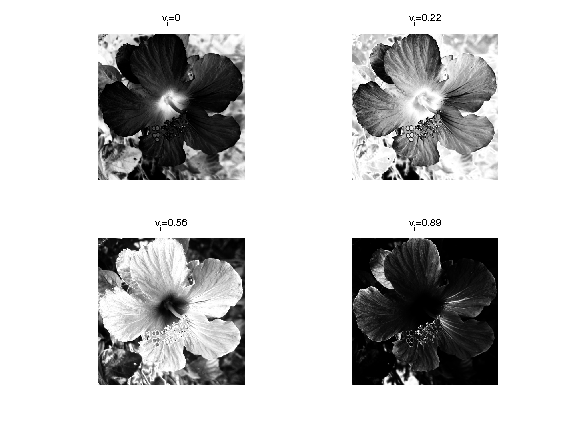In :
%% Insert your code here.


Shortcut to compute the bilateral stack $\{ F_{v_i} \}_i$.

In :
bileteral_stack_tmp = @(f0,sx,W)Filter(W.*repmat(f0, [1 1 p]), sx) ./ Filter(W, sx);
bileteral_stack = @(f0,sx,sv)bileteral_stack_tmp(f0,sx,WeightMap(f0,sv));


Compute the bilateral stack $\{ F_{v_i} \}_i$.

In :
F = bileteral_stack(f0,sx,sv);


Exercise 3

Display several stacks $F_{v_i}$.

In :
exo3()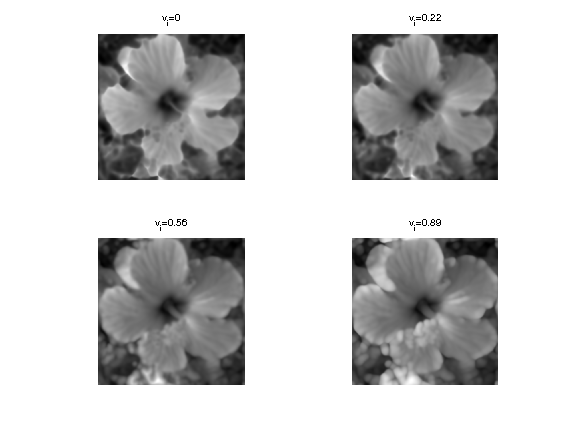In :
%% Insert your code here.


Destacking corresponds to selecting a layer $I(x) \in \{1,\ldots,p\}$ at each pixel. $$f_I(x) = F_{ v_{I(x)} }(x).$$

Shortcut for de-stacking using a set of indexes.

In :
[y,x] = meshgrid(1:n,1:n);
indexing = @(F,I)F(I);
destacking = @(F,I)indexing(F,x + (y-1)*n + (I-1)*n^2);


The simplest reconstruction method performs the destacking using the nearest neighbor value: $$I(x) = \uargmin{ 1 \leq i \leq p } \abs{f(x)-v_i}.$$

Shortcut for performing de-stacking by nearest neighbor interpolation.

In :
bilateral_nn = @(f0,sx,sv)destacking( bileteral_stack(f0,sx,sv), round( f0*(p-1) ) + 1 );


Display.

In :
fNN = bilateral_nn(f0,sx,sv);
clf;
imageplot( fNN );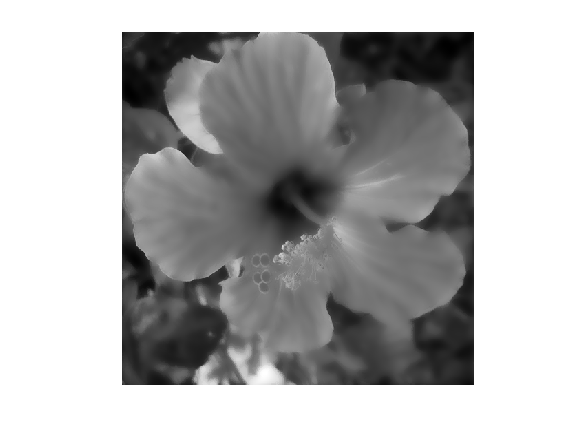A better reconstruction is obtained by using a first order linear interpolation to perform the destacking. $$\Bb_{\si_x,\si_v}(f)(x) \approx (1-\la(x))f_{I(x)}(x) + \la(x) f_{I(x)+1}(x)$$ where $I(x)$ and $\la(x)$ are defined as $$f(x) = (1-\la(x)) v_{I(x)} + \la(x) v_{I(x)+1} \qwhereq \la(x) \in [0,1).$$

Shortcut for the linear interpolation reconstruction.

In :
frac = @(x)x-floor(x);
lininterp = @(f1,f2,Fr)f1.*(1-Fr) + f2.*Fr;
bilateral_lin1 = @(F,f0)lininterp( destacking(F, clamp(floor(f0*(p-1)) + 1,1,p) ), ...
destacking(F, clamp(ceil(f0*(p-1)) + 1,1,p) ), ...
frac(f0*(p-1)) );
bilateral_lin = @(f0,sx,sv)bilateral_lin1(bileteral_stack(f0,sx,sv), f0);


Exercise 4

Compare nearest-neighbor and linear destacking.

In :
exo4()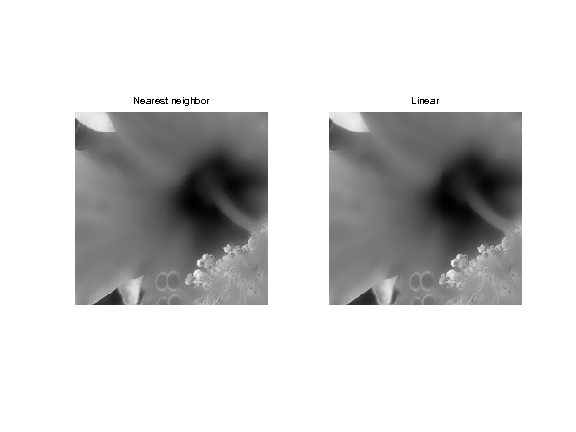In :
%% Insert your code here.


Exercise 5

Study the influence of $\sigma_x$ on the filter, for a fixed $\sigma_v=0.2$.

In :
exo5()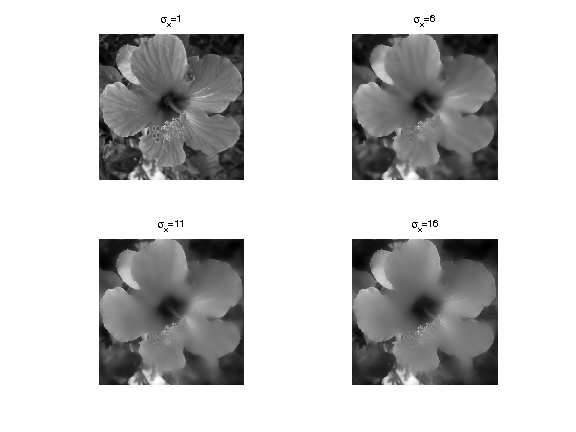In :
%% Insert your code here.


Exercise 6

Study the influence of $\sigma_v$ on the filter, for a fixed $\sigma_x=8$.

In :
exo6()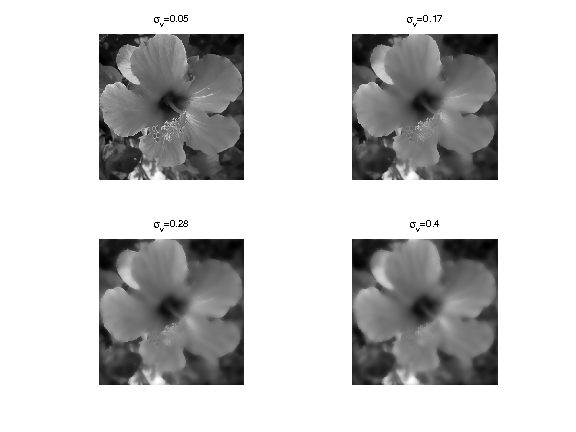In :
%% Insert your code here.


Note that this stack implementation of the bilateral filter can be quite inacurate, in particular if $p$ is small. A more precise fast implementation is described in:

A Fast Approximation of the Bilateral Filter using a Signal Processing Approach, Sylvain Paris and Fr do Durand, International Journal of Computer Vision (IJCV'09)

## Denoising using the Bilateral Filter¶

The first basic application of the bilateral filter is for denoising. It performs a filtering that respects edges.

Noise level.

In :
mu = .05;


Create a noisy image $f = f_0 + w$ where $w$ is a Gaussian white noise of variance $\mu^2$.

In :
f = f0 + randn(n,n)*mu;


Display the noisy image.

In :
clf;
imageplot(clamp(f));